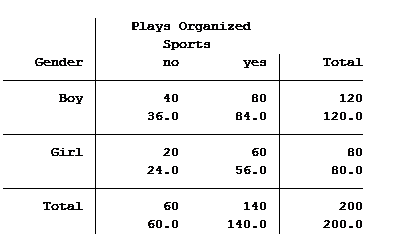# poisson

### Member Training: Logistic Regression for Count and Proportion Data

July 2nd, 2018 by

Most of us know that binary logistic regression is appropriate when the outcome variable has two possible outcomes: success and failure.There are two more situations that are also appropriate for binary logistic regression, but they don’t always look like they should be.

### Poisson or Negative Binomial? Using Count Model Diagnostics to Select a Model

March 19th, 2018 by

How do you choose between Poisson and negative binomial models for discrete count outcomes?

One key criterion is the relative value of the variance to the mean after accounting for the effect of the predictors. A previous article discussed the concept of a variance that is larger than the model assumes: overdispersion.

(Underdispersion is also possible, but much less common).

There are two ways to check for overdispersion: (more…)

### Member Training: Making Sense of Statistical Distributions

August 1st, 2017 by

Many who work with statistics are already functionally familiar with the normal distribution, and maybe even the binomial distribution.These common distributions are helpful in many applications, but what happens when they just don’t work?

This webinar will cover a number of statistical distributions, including the:

• Poisson and negative binomial distributions (especially useful for count data)
• Multinomial distribution (for responses with more than two categories)
• Beta distribution (for continuous percentages)
• Gamma distribution (for right-skewed continuous data)
• Bernoulli and binomial distributions (for probabilities and proportions)
• And more!

We’ll also explore the relationships among statistical distributions, including those you may already use, like the normal, t, chi-squared, and F distributions.

Note: This training is an exclusive benefit to members of the Statistically Speaking Membership Program and part of the Stat’s Amore Trainings Series. Each Stat’s Amore Training is approximately 90 minutes long.

### Analyzing Zero-Truncated Count Data: Length of Stay in the ICU for Flu Victims

January 9th, 2017 by

It’s that time of year: flu season.

Let’s imagine you have been asked to determine the factors that will help a hospital determine the length of stay in the intensive care unit (ICU) once a patient is admitted.

The hospital tells you that once the patient is admitted to the ICU, he or she has a day count of one. As soon as they spend 24 hours plus 1 minute, they have stayed an additional day.

Clearly this is count data. There are no fractions, only whole numbers.

To help us explore this analysis, let’s look at real data from the State of Illinois. We know the patients’ ages, gender, race and type of hospital (state vs. private).

A partial frequency distribution looks like this: (more…)

### Two-Way Tables and Count Models: Expected and Predicted Counts

December 29th, 2016 by

In a previous article, we discussed how incidence rate ratios calculated in a Poisson regression can be determined from a two-way table of categorical variables.

Statistical software can also calculate the expected (aka predicted) count for each group. Below is the actual and expected count of the number of boys and girls participating and not participating in organized sports.The value in the top of each cell is the actual count (40 boys do not play organized sports) and the bottom value is the expected/predicted count (36 boys are predicted to not play organized sports).

The Poisson model that we ran in the previous article generated the following table: (more…)

### Overdispersion in Count Models: Fit the Model to the Data, Don’t Fit the Data to the Model

December 21st, 2016 by

If you have count data you use a Poisson model for the analysis, right?

The key criterion for using a Poisson model is after accounting for the effect of predictors, the mean must equal the variance. If the mean doesn’t equal the variance then all we have to do is transform the data or tweak the model, correct?

Let’s see how we can do this with some real data. A survey was done in Australia during the peak of the flu season. The outcome variable is the total number of times people asked for medical advice from any source over a two-week period.

We are trying to determine what influences people with flu symptoms to seek medical advice. The mean number of times was 0.516 times and the variance 1.79.

The mean does not equal the variance even after accounting for the model’s predictors.

Here are the results for this model: (more…)